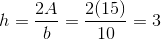## Example Questions

### Example Question #181 : Plane Geometry

If the area of an isosceles triangle isand its base is, what is the height of the triangle?Explanation:

Use the formula for area of a triangle to solve for the height: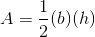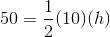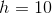### Example Question #182 : Plane Geometry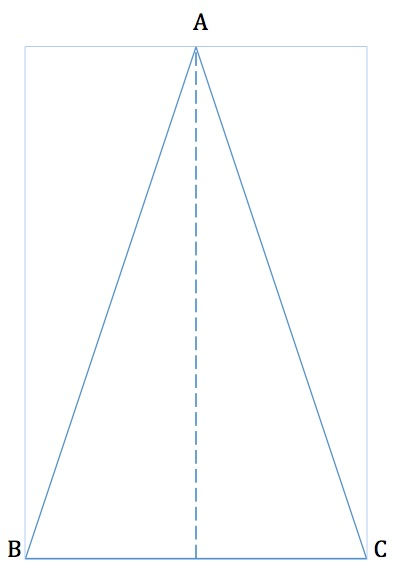Find the height of the isosceles triangle above if the length of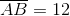and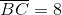. If your answer is in a decimal form, round to the nearest tenths place.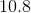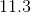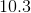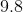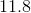Explanation:

Because this is an isosceles triangle,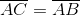. Also, we know that the base of the triangle,. Therefore, we create two triangles by bisecting the trinagle down the center. We are solving for the length of the long arm of the triangle. We know that the hypotenuse is 12 and the base is 4 (half of 8).

Thus we use the Pythagorean Theorem to find the length of the long arm: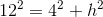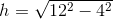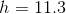### Example Question #183 : Plane Geometry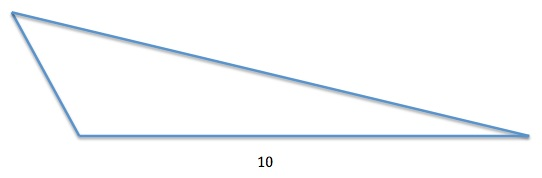The triangle above has an area ofunits squared. If the length of the base isunits, what is the height of the triangle?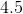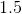Explanation:

The area of a triangle is found using the formula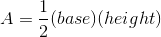The height of any triangle is the length from it's highest point to the base, as pictured below: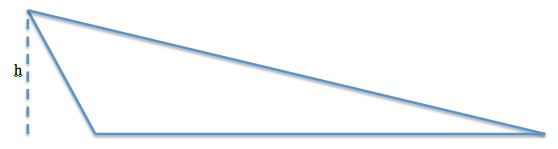We can find the height by rearranging the area formula: